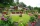Rectangle

Calculate area of the rectangle if its length is 12 cm longer than its width and length is equal to the square of its width.

Result

S =  64 cm2

Solution:Checkout calculation with our calculator of quadratic equations.

Leave us a comment of example and its solution (i.e. if it is still somewhat unclear...):Be the first to comment!To solve this verbal math problem are needed these knowledge from mathematics:

Looking for help with calculating roots of a quadratic equation?

Next similar examples:

1. Square and rectangleCalculate the side of a square which content area equals area of the rectangle having a length of 3 cm greater and by 2 cm smaller than the side of the square.
2. Rectangular garden 2A farmer bought 600 m of wire for the fence. He wants to use it to besiege a rectangular garden with a surface of 16875 m2. Calculate the size of the garden.
3. A rectangleA rectangle has an area of 36 cm2. What could the length and width of rectangle be?
4. RootsDetermine the quadratic equation absolute coefficient q, that the equation has a real double root and the root x calculate: ?
5. Variations 4/2Determine the number of items when the count of variations of fourth class without repeating is 600 times larger than the count of variations of second class without repetition.
6. Square root 2If the square root of 3m2 +22 and -x = 0, and x=7, what is m?Find the roots of the quadratic equation: 3x2-4x + (-4) = 0.
8. Theorem proveWe want to prove the sentence: If the natural number n is divisible by six, then n is divisible by three. From what assumption we started?
9. DiscriminantDetermine the discriminant of the equation: ?
10. EquationEquation ? has one root x1 = 8. Determine the coefficient b and the second root x2.
11. Expression with powersIf x-1/x=5, find the value of x4+1/x4
12. Expressions 3If k(x+6)= 4x2 + 20, what is k(10)=?
13. Evaluation of expressionsIf a2-3a+1=0, find (i)a2+1/a2 (ii) a3+1/a3
14. AlgebraX+y=5, find xy (find the product of x and y if x+y = 5)
15. GP membersThe geometric sequence has 10 members. The last two members are 2 and -1. Which member is -1/16?Which of the points belong function f:y= 2x2- 3x + 1 : A(-2, 15) B (3,10) C (1,4)Solve quadratic equation: (6n+1) (4n-1) = 3n2